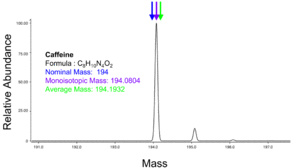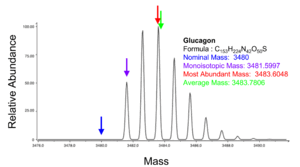# Mass (mass spectrometry)

﻿
Mass (mass spectrometry)

The mass recorded by a mass spectrometer can refer to different physical quantities depending on the characteristics of the instrument and the manner in which the mass spectrum is displayed.

## Accurate mass

The accurate mass (more appropriately, the measured accurate mass) is an experimentally determined mass that allows the elemental composition to be determined. For molecules with mass below 200 u, a 5 ppm accuracy is sufficient to uniquely determine the elemental composition.

## Average mass

The average mass of a molecule is obtained by summing the average atomic masses of the constituent elements. For example, the average mass of natural water with formula H2O is 1.00794 + 1.00794 + 15.9994 = 18.01528.

## Exact mass

The exact mass (more appropriately, the calculated exact mass) is obtained by summing the masses of the individual isotopes of the molecule. For example, the exact mass of water containing two hydrogen-1 (1H) and one oxygen-16 (16O) is 1.0078 + 1.0078 + 15.9949 = 18.0105. The exact mass of heavy water, containing two hydrogen-2 (deuterium or 2H) and one oxygen-16 (16O) is 2.0141 + 2.0141 + 15.9949 = 20.0229.

## Isotopomer

An isotopomer (isotopic isomer) is an isomer having the same number of each isotopic atom but differing in their positions. For example, CH3CHDCH3 and CH3CH2CH2D are a pair of constitutional isotopomers. An isotopomer should not be confused with isotopologues, which are chemical species that differ only in the isotopic composition of their molecules or ions. An example is water, where three of its hydrogen-related isotopologues are: HOH, HOD and DOD, where D stands for deuterium (2H).

## Kendrick mass

The Kendrick mass is a mass obtained by multiplying the measured mass by a numeric factor. The Kendrick mass is used to aid in the identification of molecules of similar chemical structure from peaks in mass spectra. The method of stating mass was suggested in 1963 by the chemist Edward Kendrick.

According to the procedure outlined by Kendrick, the mass of CH2 is defined as 14.000 Da, instead of 14.01565 Da.

The Kendrick mass for a family of compounds F is given by$Kendrick~mass~(F) = (observed~mass) \times \frac{nominal~mass~F}{exact~mass~F}~$.

For hydrocarbon analysis, F=CH2.

## Mass number

The mass number, also called the nucleon number, is the number of protons and neutrons in an atomic nucleus. The mass number is unique for each isotope of an element and is written either after the element name or as a superscript to the left of an element's symbol. For example, carbon-12 (12C) has 6 protons and 6 neutrons.

## Molecular massTheoretical isotope distribution for the molecular ion of caffeine

The molecular mass (abbreviated Mr) of a substance, formerly also called molecular weight and abbreviated as MW, is the mass of one molecule of that substance, relative to the unified atomic mass unit u (equal to 1/12 the mass of one atom of 12C). Due to this relativity, the molecular mass of a substance is commonly referred to as the relative molecular mass, and abbreviated to Mr.

## Monoisotopic mass

The monoisotopic mass is the sum of the masses of the atoms in a molecule using the unbound, ground-state, rest mass of the principal (most abundant) isotope for each element instead of the isotopic average mass. For typical organic compounds, where the monoisotopic mass is most commonly used, this also results in the lightest isotope being selected. For some heavier atoms such as iron and argon the principal isotope is not the lightest isotope. The term is designed for measurements in mass spectrometry primarily with smaller molecules. It is not typically useful as a concept in physics or general chemistry. Monoisotopic mass is typically expressed in unified atomic mass units (u), also called daltons (Da).

## Most abundant massTheoretical isotope distribution for the molecular ion of glucagon (C153H224N42O50S)

This refers to the mass of the molecule with the most highly represented isotope distribution, based on the natural abundance of the isotopes.

## Nominal mass

The nominal mass of an ion or molecule is calculated using the integer mass (ignoring the mass defect) of the most abundant isotope of each element. This is the equivalent of summing the mass numbers of all constituent atoms. For example H = 1, C = 12, O = 16, etc. The nominal mass of H2O is 18, for example.

Wikimedia Foundation. 2010.

### Look at other dictionaries:

• Mass spectrometry — (MS) is an analytical technique that measures the mass to charge ratio of charged particles. It is used for determining masses of particles, for determining the elemental composition of a sample or molecule, and for elucidating the chemical… …   Wikipedia

• Mass spectrometry software — is software used for data acquisition, analysis, or representation in mass spectrometry. Contents 1 MS/MS peptide identification 1.1 Database search algorithms 1.1.1 SEQUEST 1.1.2 …   Wikipedia

• Mass spectrometry imaging — (also known as imaging mass spectrometry) is a technique used in mass spectrometry to visualize the spatial distribution of e.g. compounds, biomarker, metabolites, peptides or proteins by their molecular masses. Emerging technologies in the field …   Wikipedia

• Mass spectrometry data format — Mass spectrometry is a scientific technique for measuring the mass of ions. It is often coupled to chromatographic techniques such as gas or liquid chromatography and has found widespread adoption in the fields of analytical chemistry and… …   Wikipedia

• Mass Spectrometry Reviews —   Titre abrégé Mass Spectrom. Rev. Discipline Chimie …   Wikipédia en Français

• Mass Spectrometry Reviews —   Abbreviated title (ISO) …   Wikipedia

• mass spectrometry — mass spectroscopy mass spectroscopy . 1. (Physics, Chemistry) A method for identifying chemical composition of substances by use of a {mass spectrometer}. [PJC] …   The Collaborative International Dictionary of English

• mass spectrometry — Spectrometry Spec*trom e*try, n. (Physics) Art or process of using a spectrometer, of any type; when used alone, it usually refers to the use of a spectrometer using light in the visible, infrared, or ultraviolet region. {Spec tro*met ric}, a.… …   The Collaborative International Dictionary of English

• mass spectrometry — n an instrumental method for identifying the chemical constitution of a substance by means of the separation of gaseous ions according to their differing mass and charge called also mass spectroscopy mass spectrometric adj mass spectrometrically… …   Medical dictionary

• mass spectrometry — or mass spectroscopy Analytic technique by which chemical substances are identified by sorting gaseous ions by mass using electric and magnetic fields. A mass spectrometer uses electrical means to detect the sorted ions, while a mass spectrograph …   Universalium